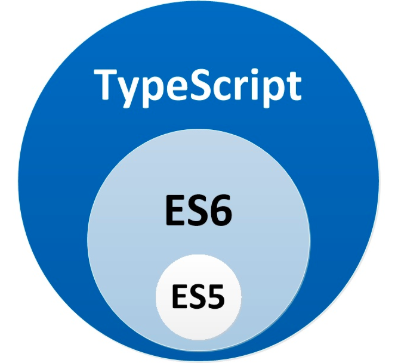# 《前端之路》 - 初试 TypeScript（一）基础数据类型

2019/04/10 10:10

[TOC]

### 一、先讲讲 TypeScript

`typeScript 是 Javascript 的超集` 我们用一张图来简单介绍下 ts 和 js 清清楚楚明明白白的关系～JS里面一切皆为对象，通过原型链来定义了系列不同类型数据的原生方法，然后给到了系列可以改变上下文的奇淫巧技，让 JS 在使用起来的时候可以非常方便，不需要考虑过多，链上了咱们就疯狂使用，这样真的好么？（ 灵魂拷问 ）

### 二、 typeScript 的数据类型 （Basic Types）

typeScript 的 Basic Types 包含了哪些呢？

• Boolean （布尔类型）
• Number （数字类型）
• String （字符串类型）
• Array （数组类型）
• Tuple （元组类型）
• Enum （可枚举类型）
• Any （任意类型）
• Void （Void 类型）
• Null & Undefined
• Never （Never 类型）
• Object （object 类型-非原始类型）
##### 2.1.1 Boolean （布尔类型）

`TypeScript` 语法

``````let isBool: Boolean = false;
``````

``````"use strict";
var isBool = false;
``````
##### 2.1.2 Number （数字类型）

`TypeScript` 语法

``````let nums: number = 123;
``````

``````"use strict";
var nums = 123;
``````
##### 2.1.3 String （字符串类型）

`TypeScript` 语法

``````let str: string = "123";
``````

``````"use strict";
var str = "123";
``````
##### 2.1.4 Array （数组类型）

`TypeScript` 语法

``````// 纯数组类型数组表示方式
let arr0: number[] = [123, 123];
// 使用数组泛型
let arr1: Array<number> = [123, 345];
let arr2: Array<string> = ["123", "345"];
let arr3: Array<any> = [123, "345", { title: "123" }];
``````

``````"use strict";
// 纯数组类型数组表示方式
var arr0 = [123, 123];
// 使用数组泛型
var arr1 = [123, 345];
var arr2 = ["123", "345"];
var arr3 = [123, "345", { title: "123" }];
``````
##### 2.1.5 Tuple （元组类型）
``````稍微解释下什么叫元组类型,元组类型本质上也属于 数组类型的一种，是数组类型的子集，然后不同的一点在于，元组类型的数据长度是已知的。就是说我们为这里每一位数组中的数据定义类型。那这种类型就叫 ##元组类型##
``````

`TypeScript` 语法

``````let tupleArr: [number, string];
tupleArr = [123, "123"];
``````

``````"use strict";
var tupleArr;
tupleArr = [123, "123"];
``````
##### 2.1.6 Enum （可枚举类型）
``````稍微解释下什么叫可枚举类型，因为这对于大多数只了解 JS 的前端开发者来讲是一个新的数据类型，但是 `可枚举类型` 在 c/c#/java 中已经是非常常见的了。一句话概括就是，枚举是组织收集有关联变量的一种方式。来，我们先举个🌰：

在 c语言中，我们需要定义一系列的变量，我们一般会这样去定义：
``````
``````#define MON 1
#define TUE 2
#define WED 3
#define THU 4
.
.
.
``````
``````如果有了枚举类型，我们又可以怎么来定义呢？
``````
``````enum DAY {
MON=1, TUE, WED, THU, FRI, SAT, SUN
}
``````

`TypeScript` 语法

``````enum HttpStutas {
success = 200,
error = 404,
noRequestId = 10010,
noRequestName = 10011,
}

let res = HttpStutas.success;
console.log(res);
``````

``````"use strict";
var HttpStutas;
(function (HttpStutas) {
HttpStutas[HttpStutas["success"] = 200] = "success";
HttpStutas[HttpStutas["error"] = 404] = "error";
HttpStutas[HttpStutas["noRequestId"] = 10010] = "noRequestId";
HttpStutas[HttpStutas["noRequestName"] = 10011] = "noRequestName";
})(HttpStutas || (HttpStutas = {}));
var res = HttpStutas.success;
console.log(res);	// 200
console.log(HttpStutas)
/**
* { '200': 'success',
'404': 'error',
'10010': 'noRequestId',
'10011': 'noRequestName',
success: 200,
error: 404,
noRequestId: 10010,
noRequestName: 10011 }
*
*/

// 这里对于我们需要的一个理解是，在 JavaScript 赋值运算符，就是这里的等号，返回的解决是被赋的这个值。

// 这里其实我们就简单的定义了一些 http 请求返回的状态码，我们实时根据状态码来转译成我们可以轻松看懂的字符串，这里也算是一个前端的同学在日常开发中常常会遇到的问题
``````
##### 2.1.7 Any （任意类型）

`TypeScript` 语法

``````let a: any = '123';
a = 123;
a = true;
a = {
x: '123',
};

let arr: any[] = [1, '123', {}, true, ];
``````

``````"use strict";
var a = '123';
a = 123;
a = true;
a = {
x: '123',
};
var arr = [1, '123', {}, true, ];
``````
##### 2.1.8 Viod （Viod类型）
``````这里也需要简单介绍了，一般我们的函数一般其实会分为2种，一种是执行以后有数据返回的，一种是没有任何数据返回的，那么在介绍 Viod 之前我们就需要介绍下，函数确认返回数据类型的时候，我们需要怎么去写。
``````

`TypeScript` 语法

``````let fun1 = function(): string {
return '123';
};

let fun2 = function(): number {
return 123;
};

let fun3 = function(): Array<number> {
return [1, 213, 213];
};

let fun4 = function(): Array<string> {
return ['13', 'axa'];
};

let fun5 = function(): Array<any> {
return ['13', 213, {}];
};

let fun6 = function(): any {
return { a: 123 };
};

let fun7 = function(): void {
console.log(fun1);
};

// 注意这里的 fun7 其实就是没有 return 任何数据，这个时候我们就给这个function 定义成一个 viod 类型。
``````

``````"use strict";
var fun1 = function () {
return '123';
};
var fun2 = function () {
return 123;
};
var fun3 = function () {
return [1, 213, 213];
};
var fun4 = function () {
return ['13', 'axa'];
};
var fun5 = function () {
return ['13', 213, {}];
};
var fun6 = function () {
return { a: 123 };
};
var fun7 = function () {
console.log(fun1);
};
``````
##### 2.1.9 null & undefined （null 和 undefied 类型）

`TypeScript` 语法

``````let n: null = null;
let u: undefined = undefined;

console.log(n === null); // true
``````

``````"use strict";
var n = null;
var u = undefined;
console.log(n === null); // true
``````
##### 2.1.10 never（never 类型）

`TypeScript` 语法

``````let ass: never;

// ass = 123; // ts 会报错

// never 是 null 和 undefined 的子集，表示从来不会出现的类型

ass = (() => {
// ts 正确写法
throw Error('错误');
})();

// 但是往往，我们用never类型比较少，一般会用 number 类型或者 string 类型替代

``````

``````"use strict";
var ass;
// ass = 123; // ts 会报错
// never 是 null 和 undefined 的子集，表示从来不会出现的类型
ass = (function () {
// ts 正确写法
throw Error('错误');
})();
// 但是往往，我们用never类型比较少，一般会用 number 类型或者 string 类型替代
``````
##### 2.1.11 object （object 类型）
``````其实这里简单解释一下，什么是 object 类型，object 是非原始类型，且是 非 number, string, boolean, symbol, null, or undefined. 以外的一种类型
``````

`TypeScript` 语法

``````let o: object;
let names: string = 'zhang';
o = {
names,
};
``````

``````"use strict";
var o;
var names = 'zhang';
o = {
names: names,
};
``````

## 这篇文章，总结来说是先介绍了下 typeScript 的基础数据类型，以及 typeScript 的 what、why、how 的一个基本介绍，介绍 什么是 typeScript，为什么要用typeScript以及 如何使用 typeScript 。那么我们初次接触到的话，主要是需要注意 语法上的差异，因为往往，我们在给各种数据类型定义类型的时候往往会出现一些语法错误，这里大家在书写的时候注意一下，如果出错可以通过 vscode 查看对应的错误提示.

GitHub 地址：（欢迎 star 、欢迎推荐 : ) 《前端之路》 - 初试 TypeScript（一）基础数据类型

0
0 收藏

### 作者的其它热门文章0 评论
0 收藏
0